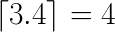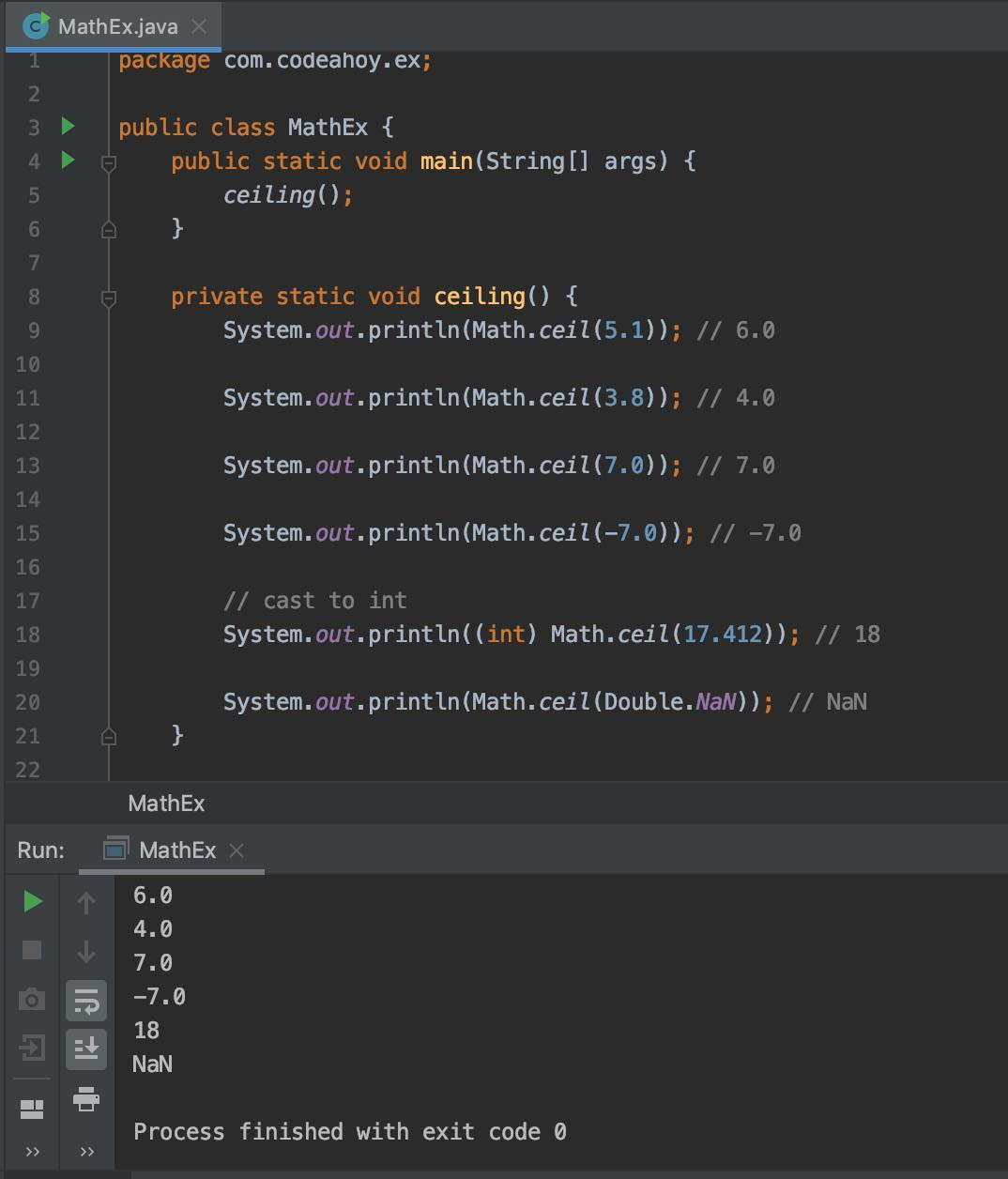# Java Math.ceil() Method with Examples

Oct 12, 2019 · 2 mins readThe java.lang.Math class that comes bundled with Java contains various methods for performing basic numeric operations such as the elementary exponential, logarithm, square root, and trigonometric functions.

Math.ceil(double) method is part of the Math class. We use it to find the ceiling value of a number. This function returns the largest integer greater than or equal to the argument provided. In other words, it returns the next largest integer value of the specified number. For example, if you pass it 3.4, it will return 4.Let’s look at its method signature:

``````public static double ceil(double a)
``````

It takes an argument of type `double` and returns a value as a `double` that is less than or equal to the argument and is equal to a mathematical integer.

``````System.out.println(Math.ceil(4.1)); // 5.0
``````

## Code Example

The following example illustrates how to use Math.ceil(double) method.

``````public class MathEx {
public static void main(String[] args) {
ceiling();
}

private static void ceiling() {
System.out.println(Math.ceil(5.1)); // 6.0

System.out.println(Math.ceil(3.8)); // 4.0

System.out.println(Math.ceil(7.0)); // 7.0

System.out.println(Math.ceil(-7.0)); // -7.0

// cast to int
System.out.println((int) Math.ceil(17.412)); // 18

System.out.println(Math.ceil(Double.NaN)); // NaN
}
}
``````

Output

``````6.0
4.0
7.0
-7.0
18
NaN
``````

Here’s a screenshoot of the code above if you’re on mobile and having trouble reading the code above.### Special cases

• If the argument value is already equal to a mathematical integer, then the result is the same as the argument.
• If the argument is NaN or an infinity or positive zero or negative zero, then the result is the same as the argument.
• If the argument value is less than zero but greater than -1.0, then the result is negative zero.

If you need more detailed information, please see Javadocs. It’s worth noting that the `Math` class in Java contains several other useful methods for arithmetic, logarithms and trignometric operations.

#### You May Also Enjoy

If you like this post, please share using the buttons above. It will help CodeAhoy grow and add new content. Thank you!